# Simulate Probabilistic Long-Term Effects in Models with Temporal Dependence

Calculates and depicts probabilistic long-term effects in binary models with temporal dependence variables. The package performs two tasks. First, it calculates the change in the probability of the event occurring given a change in a theoretical variable. Second, it calculates the rolling difference in the future probability of the event for two scenarios: one where the event occurred at a given time and one where the event does not occur. The package is consistent with the recent movement to depict meaningful and easy-to-interpret quantities of interest with the requisite measures of uncertainty. It is the first to make it easy for researchers to interpret short- and long-term effects of explanatory variables in binary autoregressive models, which can have important implications for the correct interpretation of these models.in models with temporal dependence

Christopher Gandrud and Laron K. Williams

Version: 1.0pltesim implements Williams's (2016) method for simulating probabilistic long-term effects in models with temporal dependence.

It is built on the coreSim package.

# Process

To find and show probabilistic long-term effects in models with temporal dependence with pltesim:

1. Estimate the coefficients. Currently pltesim works with binary outcome models, e.g. logit, so use `glm` from the default R installation.

2. Create a data frame with your counterfactual. This should have a row with the fitted counterfactual values and columns with names matching those in your fitted model. All variables without values will be treated as 0 in the counterfactual.

3. Simulate the long-term effects with `plte_builder`.

4. Plot the results with `plte_plot`.

# Examples

These examples replicate Figure 1 in Williams (2016). First estimate your model. You may need to use `btscs` to generate spells for the binary dependent variable.

``````library(pltesim)
library(ggplot2)

data('negative_year')
neg_set <- btscs(df = negative_year, event = 'y', t_var = 'year',
cs_unit = 'group', pad_ts = FALSE)

# Estimate the model
m1 <- glm(y ~ x + spell_time + I(spell_time^2) + I(spell_time^3),
data = neg_set)
``````

Then fit the counterfactual:

``````counterfactual <- data.frame(x = 0.5)
``````

Now simulate and plot long-term effects for a variety of scenarios using `plte_builder` and `plte_plot`. `plte_builder` takes as its input the fitted model object with the estimated coefficients (`obj`), an identification of the basic time period variable (`obj_tvar`), the counterfactual (`cf`), how long the counterfactual persists (`cf_duration`, it is `permanent` by default), and the time period points over which to simulate the effects.

Note that by default the predicted probabilities from logistic regression models are found. You can specify a custom quantity of interest function with the `FUN` argument.

In this first example the counterfactual is persistent throughout the entire time span:

``````# Permanent
sim1 <- plte_builder(obj = m1, obj_tvar = 'spell_time',
cf = counterfactual, t_points = c(13, 25))

plte_plot(sim1) + ggtitle('Permanent')
``````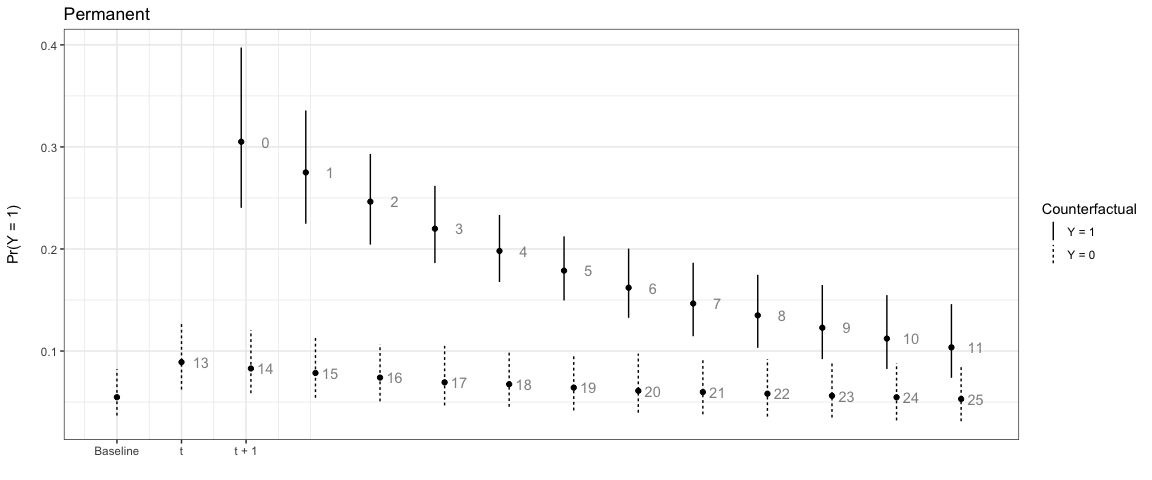Note that the numbers next to each simulation point indicate the time since the last event. You can choose to not show these numbers by setting `t_labels = FALSE` in the `plte_plot` call.

In the next example, the effect only lasts for one time period:

``````# One-time
sim2 <- plte_builder(obj = m1, obj_tvar = 'spell_time', cf_duration = 'one-time',
cf = counterfactual, t_points = c(13, 25))

plte_plot(sim2) + ggtitle('One-time')
``````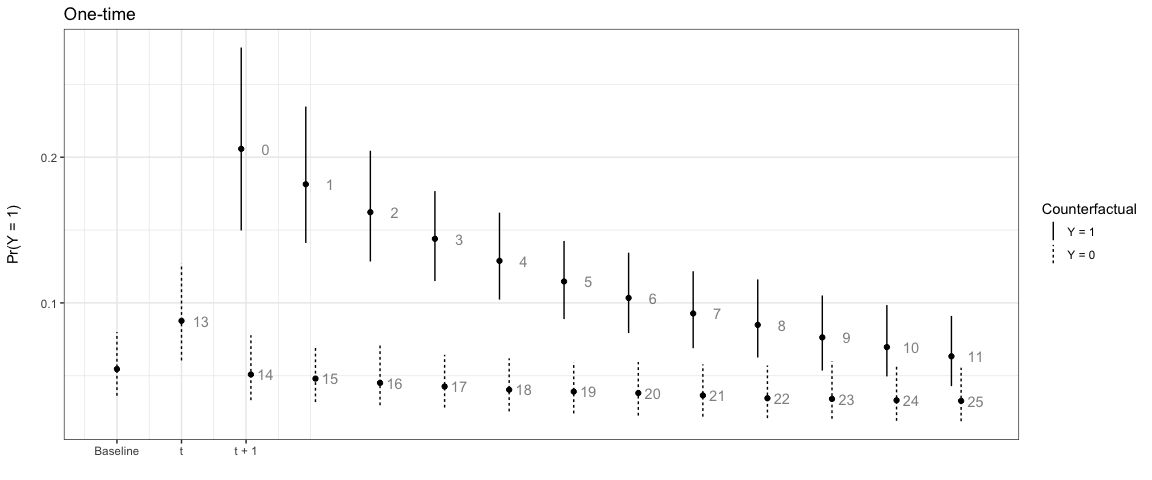We can also have the counterfactual effect last for short periods of time and simulate the effect if another event occurs:

``````# Temporary
sim3 <- plte_builder(obj = m1, obj_tvar = 'spell_time', cf_duration = 4,
cf = counterfactual, t_points = c(13, 25))

plte_plot(sim3) + ggtitle('Temporary')
``````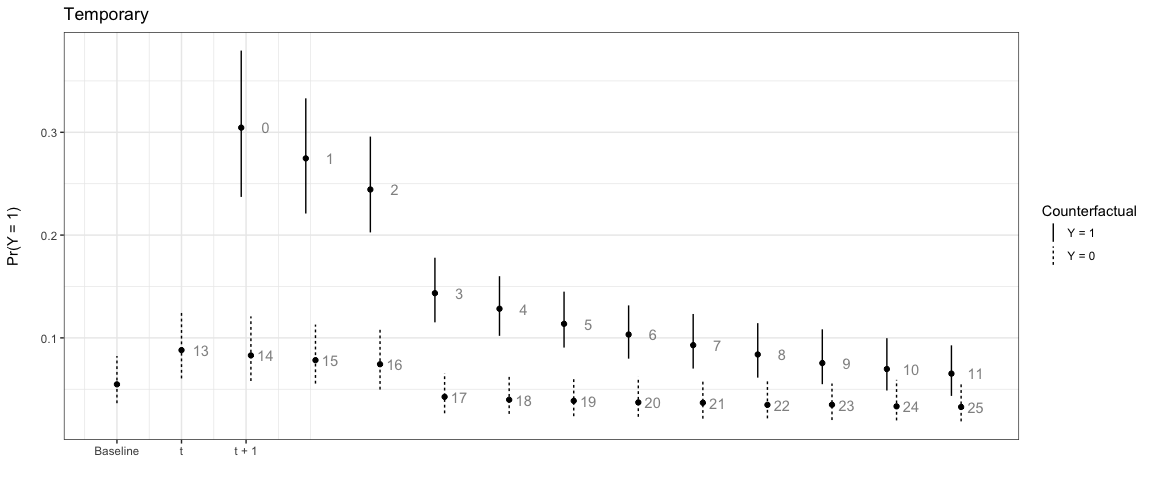``````# Multiple events, permanent counter factual
sim4 <- plte_builder(obj = m1, obj_tvar = 'spell_time',
cf = counterfactual, t_points = c(13, 20, 25))

plte_plot(sim4) + ggtitle('Permanent, Multiple Events')
``````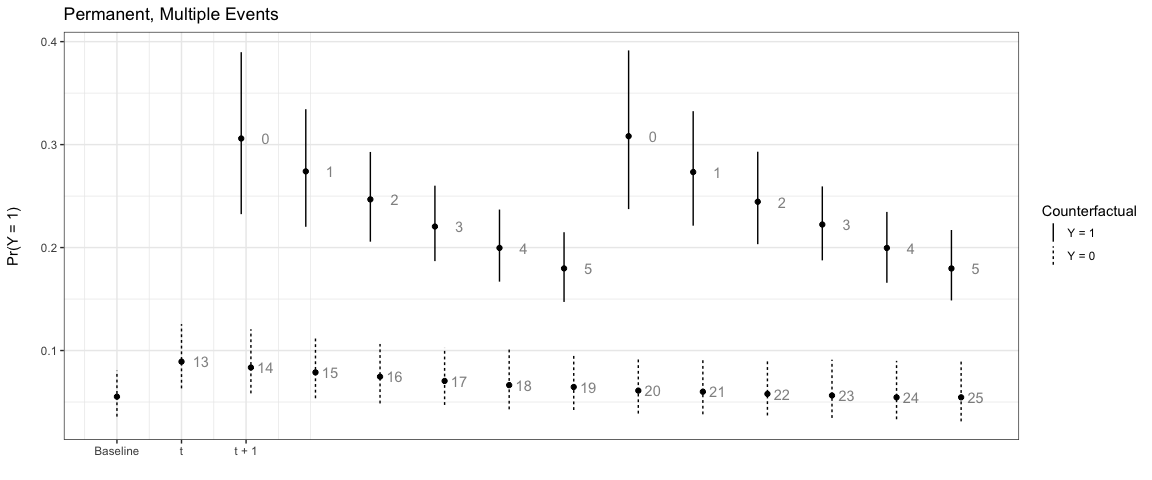By default the baseline scenario has all covariate values fitted at 0. You can supply a custom baseline scenario in the second row of the counterfactual (`cf`) data frame. For example:

``````# Custom baseline scenario
counterfactual_baseline <- data.frame(x = c(1, 0.5))

sim5 <- plte_builder(obj = m1, obj_tvar = 'spell_time', cf_duration = 4,
cf = counterfactual_baseline, t_points = c(13, 25))

plte_plot(sim5) + ggtitle('Temporary, Custom Baseline')
``````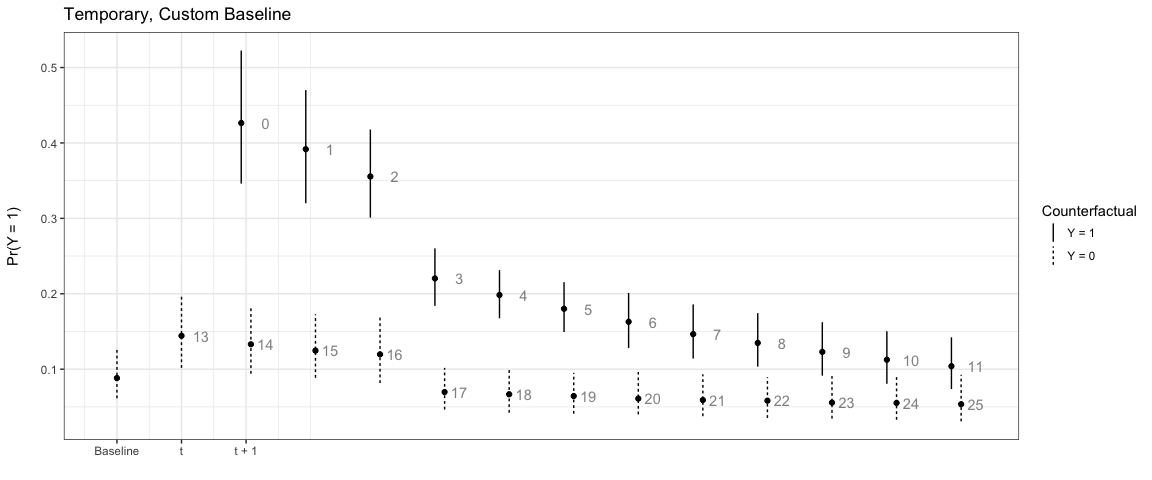# Version 1.0

• !!Breaking change!!--`btscs` now starts the spell time counter from 1, rather than 0. The returned variable is also call `spell_time` rather than `spell` as the former is more intuitive.

• `btscs` checks that all supplied variables are in `df`. Error otherwise.

• Includes a modified version of the `negative` data set called `negative_year`. This enables more intuitive examples.

# Version 0.1.2

• Linetype for `plte_plot` now matches Williams (2016), e.g. solid lines for Y = 1.

• Minor documentation improvements.

# Version 0.1.1

• `plte_builder` finds polynomial splines using the B-spline basis. Note: apart from the degree of the piecewise polynomial, the default behaviour for `bs` is assumed.

• Added ability to supply a custom baseline scenario to `plte_builder via the`cf` argument.

# Version 0.1.0

• Minimal working version.

# Reference manual

install.packages("pltesim")

1.0 by Christopher Gandrud, 3 years ago

https://CRAN.R-project.org/package=pltesim

Report a bug at https://github.com/christophergandrud/pltesim/issues

Browse source code at https://github.com/cran/pltesim

Authors: Christopher Gandrud [aut, cre] , Laron K. Williams [aut]

Documentation:   PDF Manual